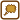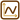## Little Paulie

Little Paulie started off on a bike on a straight road. The acceleration dependence on time is given by the following table:

 t / 10-1s 0 1 2 3 4 5 6 7 8 9 10 a / m·s-2 4,0 3,7 3,3 3,0 2,6 2,3 1,9 1,6 1,2 0,8 0,4

Draw a graph and determine the shape of the function a(t). Determine the course of Paulie’s speed and position dependence on time from it.

Suppose that Paulie was not moving at time t = 0 s and was at the origin of the coordinate system.

Comment: We should speak about the absolute value of acceleration and the absolute value of speed to be more precise. The movement is one-directional and its direction is not changing, thus we will speak about acceleration and speed.

• #### Hint 1: Graph of Paulie’s acceleration dependence on time

Draw a graph of acceleration dependence on time from the given table.

What is the graph of the given dependence?

• #### Hint 2: Expressing the function a(t)

Use the slope-intercept form of a line to express the function a(t).

• #### Hint 3: Paulie’s speed time dependence

You know how Paulie’s speed changes with time. How to you find out his speed dependence on time?

• #### Hint 4: Paulie’s position course

You already know the time dependence of Paulie’s speed.

How do you find his position dependence on time?

• #### Solution

Comment: Equations should be written for example as:

$y\,=\,1\,\mathrm{m}-2\,\mathrm{m \cdot s^{-1}}\cdot t\,.$

We don’t write units for simplification.

We will draw a graph of Paulie’s acceleration dependence on time according to the table:

The graph is a line.

We will use the slope-intercept form of a line to express the function a(t):

$a\left(t\right)\,=\,-kt+q\,,$

$$q\, =\, 4$$  (intersection with the vertical axis)

$$k\,=\,tg\,\alpha$$ (slope)

$tg\alpha\,=\,\frac{\Delta{a}}{\Delta{t}} \,=\, \frac{4-0{,}4}{1} \,=\, 3{,}6\,.$

( α is the angle that the line clamps with the x-axis)

Therefore: $a\left(t\right)\,=\,-3{,}6t+4\,.$

The course of Paulie’s speed can be found by integration of a(t):

$v\left(t\right)\,=\,\int{a\left(t\right)}dt\,=\,\int{\left(-3{,}6t+4\right)}dt\,,$ $v\left(t\right)\,=\,-\frac{3{,}6}{2}t^{2}+4t+C\,=\,-1{,}8^{2}+4t+C\,.$

We can find the constant C from the initial conditions:

for t = 0 s is v = 0 m s-1,

thus C = 0.

We get:

$v\left(t\right)\,=\,-1{,}8t^{2}+4t\,.$

The course of Pavlík’s speed can be found by integration of v(t):

$s\left(t\right)\,=\,\int{v\left(t\right)}dt\,=\,\int{\left(-1{,}8t^{2}+4t\right)}dt\,=\, -\frac{1{,}8}{3}t^{3}+\frac{4}{2}t^{2}+D\,,$ $s(t)\,=\, -0{,}6t^{3}+2t^{2}+D\,.$

We can find the constant D from the starting conditions:

for t = 0 s is s = 0 m,

thus D = 0.

Therefore: $s\left(t\right)\,=\,-0{,}6t^{3}+2t^{2}\,.$

The graph of Paulie’s acceleration dependence on time:

The function a(t) is expressed as:

$a\left(t\right)\,=\,-3{,}6t+4\,.$

The course of speed v(t) is:

$v\left(t\right)\,=\,-1{,}8t^{2}+4t\,.$

The course of position s(t) is:

$s\left(t\right)\,=\,-0{,}6t^{3}+2t^{2}\,.$×Original source: Mandíková,D., Rojko, M.: Soubor úloh z mechaniky pro studium učitelství. I. část. Interní materiál, MFF UK, Praha 1994 - upraveno Zpracováno v diplomové práci Jany Moltašové (2011).